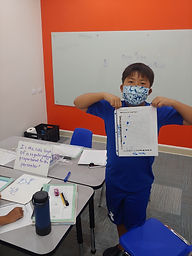## Ms. Mari

### Target 1​

###### Lesson Type:

Review

Data Probability

:

Ratio

Use ratio reasoning to calculate and compare unit price.

###### 1:

Use proportional reasoning to calculate the actual cost of individual items.

###### 2:

Use percentages to determine the price per unit when a discount is applied.

6th

###### Vocabulary:

UNIT RATE, RATIO, PROPORTION, PERCENT DISCOUNT

Activities:

- When given two rates (\$/item), students determined which item was "better buy".

- Also, students made comparisons between unit rates when a percentage discount was also applied.### Home Exploration

###### Guiding Questions:## Absent Students:

Mikayla

### Target 2

:

###### 1:

Determine whether quantities are in a proportional relationship.

###### 2:

Understand that proportional relationships create a straight line.

###### 3:

Test for equivalent ratios in a table or by graphing on the coordinate plane.

###### 4:

Use proportional relationships to solve real-world problems.

###### Vocabulary:

PROPORTIONAL RELATIONSHIP, RATIO, REGULAR POLYGON

Activities:

- Each student chose a different regular polygon (square, hexagon, octagon) and created a table comparing various side lengths on their shape to its perimeter.

- They used the table to create a graph of the data and observed that the points formed a line beginning at (0,0).

- They examined the ratios (perimeter/side length) and determined the relationship was proportional because all ratios were equal.

- They wrote an equation to describe the relationship between side length and perimeter.### Home Exploration

###### Guiding Questions:### Target 3

:

###### Vocabulary:

Activities:### Home Exploration